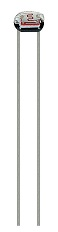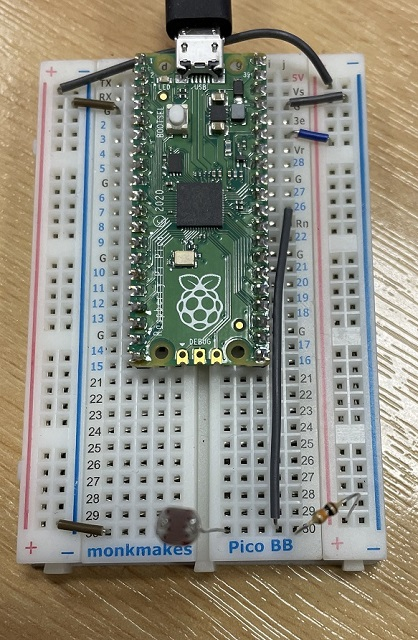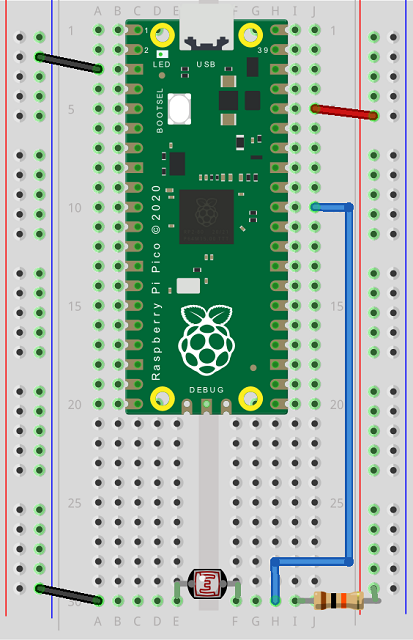# Raspberry Pi Pico Light Dependent Resistor

A light dependent resistor is a resistor which varies depending on the amount of light that is illuminating it. The one used on this page costs about 50p to buy and looks like this.To read values from the photocell (LDR), you need to make a voltage divider circuit. You do this by placing two resistors in series and reading from the connection between them. One of those resistors is the LDR, the other one is a 10KOhm (or something from 1K-10K) resistor. Connect one leg of the LDR to GND. Make two connections to the other leg. One of them to our microcontroller pin (I am using GP26) and the other to 3V using the 10K resistor.

Here is a photograph of my circuit,The Fritzing diagram for this is as follows,With the circuit setup, the programming is quite simple,

```from machine import ADC
from time import sleep